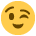#### Howdy, Stranger!

It looks like you're new here. If you want to get involved, click one of these buttons!

Supported by

# JASP and SPSS Mann Whitney p-values differ

Hello,

I am curious if anyone else gets different results for Mann Whitney U tests on JASP and SPSS. For me, both the p-values and test statistics are coming out differently (although they are close in most cases). Does anyone know why this might be?

It looks like JASP is printing out Wilcoxin (W) statistics when you check the 'Mann-Whitney U' option under independent samples t-tests.

Thanks,
Andrew

• Hi Andrew,
This issue came up before I think. Let me pass this on to the JASP team member responsible for the nonparametric tests.
Cheers,
E.J.

• Great, thank you!

• Hi Andrew,
I just took a look at this discrepancy, and what I found was that it is a general difference between SPSS and R in how they calculate the statistic.
First off, The Mann-Whitney U and the Wilcoxon Rank Sum test are the same (Wilcoxon developed the basis for the test, and Mann and Whitney expanded it). The general test procedure consists of ranking both groups together, and then summing up the ranks of one group. Sometimes, a term of $\frac{n*(n+1)}{2}$ gets subtracted from the sum, which might be a cause for differing test statistics. However, this should not affect the p-value.
Another factor that might affect the value of the W statistic is which ranks are summed: those of group 1 or group 2? In R, the sum is over those ranks that provide the greatest value, so if the sum of the ranks in group 1 is greater, that will be used as the W statistic. Of course, the two possibilities are complementing each other and again, it should not affect the p-value.
There are three factors that could influence the p-value, and might explain the difference between R output and SPSS output. The first one is the matter of continuity correction of the p-value (the test statistic is discrete, but treated as continuous, so this would correct for that). The second factor is whether the exact p-value is calculated or if an approximation is used. Thirdly, and perhaps most important, is how both programs deal with ties in the data. I do not know how SPSS does it, but R does it as follows:
say we would like to rank the numbers 1.5, 1.8, 1.8, 2.5, then in the wilcox.test() function in R, they are ranked as follows: 1, 2.5, 2.5, 4. So the ranks 2 and 3 are averaged and divided over the tied values.
I hope this clarifies things somewhat for you - maybe it would have been easier and more straightforward if there was an error somewhere in the codeKind regards,
Johnny

• Hi Johnny,
Thank you for your detailed response. This helps clarify the potential cause of the difference. SPSS gives exact p-values that are not corrected for ties; only the asymptotic p-values are corrected, so this is certainly one of the causes. The p-values are most similar between JASP and the asym. p-value of SPSS, but if I understand correctly, JASP is giving an exact p-value instead of an approximate. SPSS's method of correcting for ties seems to be the same, but it's only applied to the approximate p-value.

Thanks again for your help clearing this up.

Andrew

• Hi Andrew,
Can you help me. I have a problem with JASP. In my excel file I have four groups of participants but when I open it from JASP I don't have only one group . What can I do to resolve this problem?